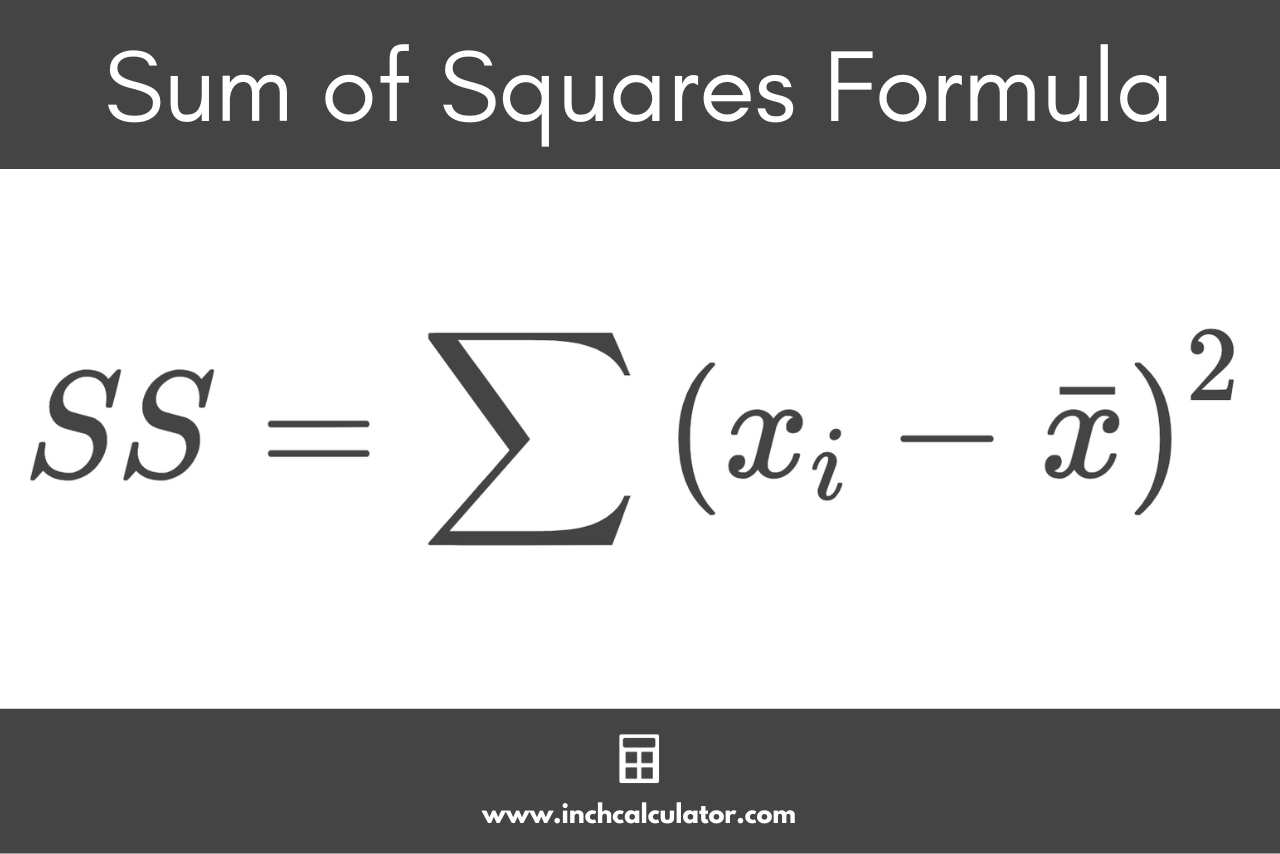# Sum of Squares Calculator

Enter the numbers in a data set to calculate the sum of squares using the calculator below.

Separate numbers using a comma (,)

## Results:

### Steps to Solve

#### Sum of Squares Formula

SS = \sum \left ( x_{i}-\bar{x} \right )^{2}

#### Step One: Find the Mean

The mean is equal to the sum of each observation xi divided by the sample size n

\bar{x} = \frac{\sum_{i=1}^{n}x_{i}}{n}

#### Step Two: Find the Deviation From the Mean

The deviation from the mean for each observation is equal to its value minus the mean .

\text{deviation} = x_{i}-\bar{x}

#### Step Three: Square the Deviations

Square each deviation from the mean.

\text{squared deviation} = \left ( x_{i}-\bar{x} \right )^{2}

#### Step Four: Find the Sum of Squares

Add each squared deviation to find the sum of squares.

SS = \sum \left ( x_{i}-\bar{x} \right )^{2}
Learn how we calculated this below

## How to Calculate the Sum of Squares

The sum of squares is a statistical measure of the deviation from the mean for numbers in a data set. The sum of squares is equal to the sum of the squares of the differences from the mean for each number or data point in the set.

The sum of squares is often used to find the variance or standard deviation in a data set.

### Sum of Squares Formula

The formula to calculate the sum of squares is:

SS = \sum \left ( x_{i}-\bar{x} \right )^{2}

Thus, the sum of squares SS is equal to the sum of each number in the set xi minus the mean squared.You can find the sum of squares in four easy steps using this formula.

### Step One: Calculate the Mean

The first step to finding the sum of squares is to find the mean. The mean is equal to the sum of all numbers in the set divided by the count of numbers in the set.

\bar{x}=\frac{\text{sum}}{\text{count}}

The mean is equal to the sum of each number in the set divided by the count.

### Step Two: Calculate the Deviations From the Mean

The next step is to calculate the deviation of each data point from the mean.

\text{deviation} = x_{i}-\bar{x}

### Step Three: Square Each Deviation

Then, calculate the square for each deviation.

\text{squared deviation} = \left ( x_{i}-\bar{x} \right )^{2}

### Step Four: Calculate the Sum of Squares

Finally, you can use the sum of squares formula to calculate it by adding the squared deviation scores for each data point together.

SS = \sum \left ( x_{i}-\bar{x} \right )^{2}

For example, let’s find the sum of squares for the numbers [1,3,4,5,8].

We’ll use the six steps for the SD calculation shown above.

Step One: Find the mean by dividing the sum of all of the values by the sample size.

\bar{x}=\frac{1+3+4+5+8}{5}
\bar{x}=\frac{21}{5}=4.2

Step Two: Subtracting the mean from each observation to find the deviations.

Observation Deviation from
1 1 – 4.2 = -3.2
3 3 – 4.2 = -1.2
4 4 – 4.2 = -0.2
5 5 – 4.2 = 0.8
8 8 – 4.2 = 3.8

Step Three: Square each of the deviations by raising it to the power of 2.

Deviation Squared Deviation
-3.2 -3.2² = 10.24
-1.2 -1.2² = 1.44
-0.2 -0.2² = 0.04
0.8 0.8² = 0.64
3.8 3.8² = 14.44

Step Four: Find the sum of squares by adding up the squared deviations.

SS = 10.24+1.44+0.04+0.64+14.44
[formula may scroll beyond screen]
SS = 26.8

So, the sum of squares for this sample is equal to 26.8